# Motion Distance and Displacement Speed and Velocity Acceleration

• Slides: 24Motion Distance and Displacement Speed and Velocity Acceleration Momentum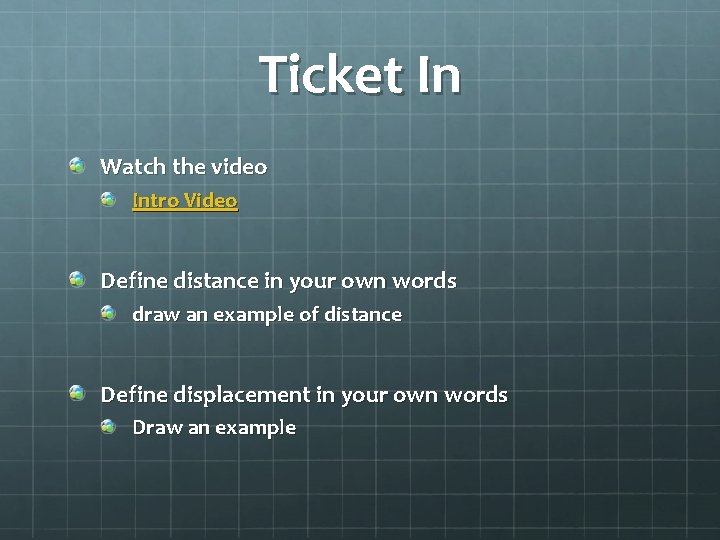Ticket In Watch the video Intro Video Define distance in your own words draw an example of distance Define displacement in your own words Draw an exampleDistance The length of a path between two points SI Unit = Meters (m) Large Distances = Kilometers (km) Small Distances = Centimeters (cm) 1 000 m = 1 km 1 m = 100 cm Example: Rollercoaster Measure path car travels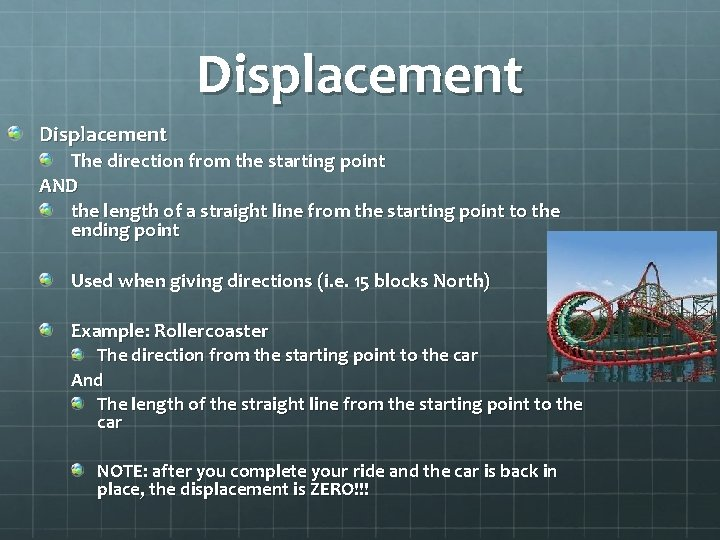Displacement The direction from the starting point AND the length of a straight line from the starting point to the ending point Used when giving directions (i. e. 15 blocks North) Example: Rollercoaster The direction from the starting point to the car And The length of the straight line from the starting point to the car NOTE: after you complete your ride and the car is back in place, the displacement is ZERO!!!Distance/Displacement Demo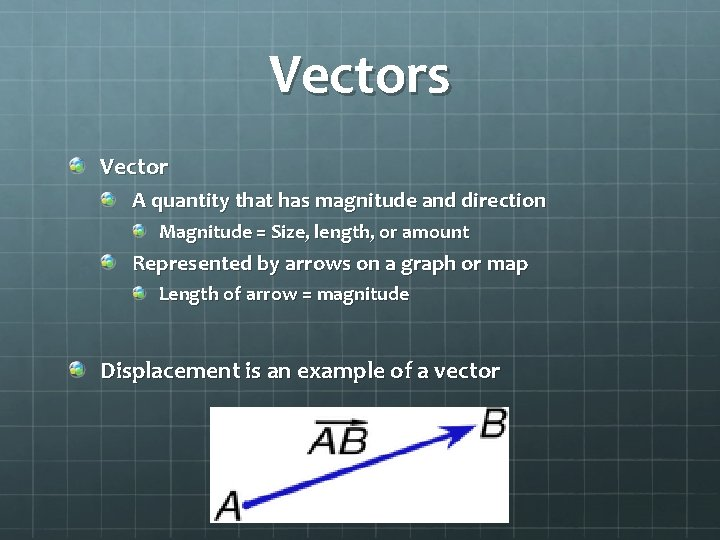Vectors Vector A quantity that has magnitude and direction Magnitude = Size, length, or amount Represented by arrows on a graph or map Length of arrow = magnitude Displacement is an example of a vectorCombining Displacements Two displacements (represented by two vectors) SAME DIRECTION OPPOSITE DIRECTION ADD MAGNITUDE SUBTRACT MAGNITUDE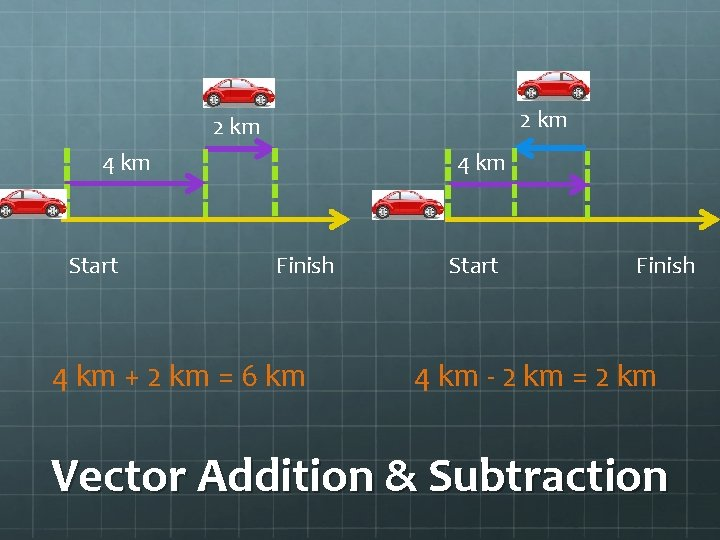2 km 4 km Start 4 km Finish 4 km + 2 km = 6 km Start Finish 4 km - 2 km = 2 km Vector Addition & SubtractionSample Problems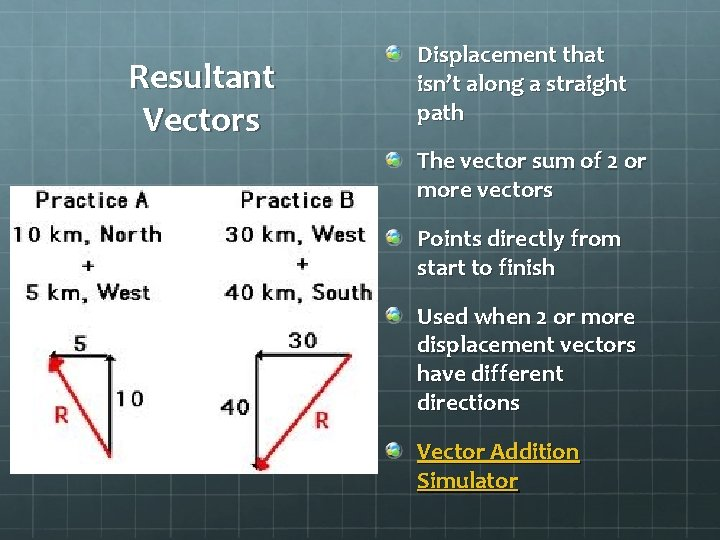Resultant Vectors Displacement that isn’t along a straight path The vector sum of 2 or more vectors Points directly from start to finish Used when 2 or more displacement vectors have different directions Vector Addition Simulator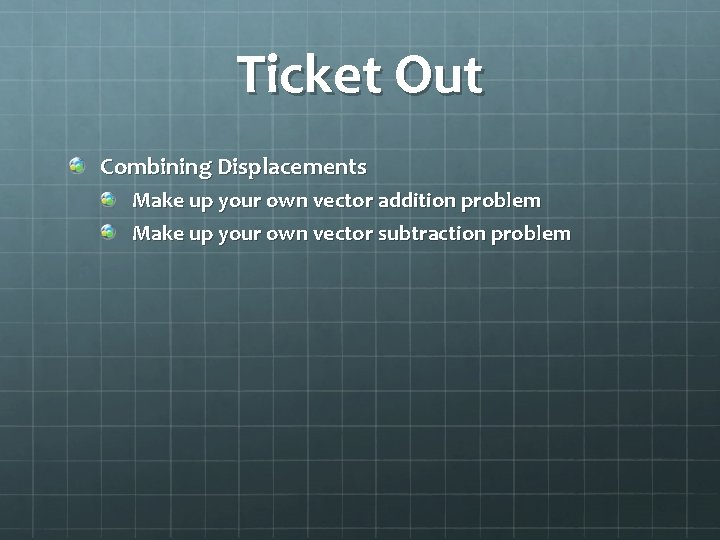Ticket Out Combining Displacements Make up your own vector addition problem Make up your own vector subtraction problem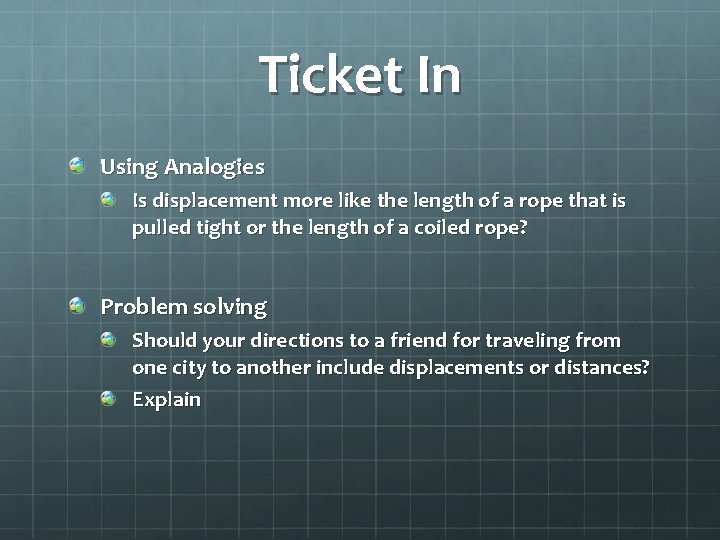Ticket In Using Analogies Is displacement more like the length of a rope that is pulled tight or the length of a coiled rope? Problem solving Should your directions to a friend for traveling from one city to another include displacements or distances? Explain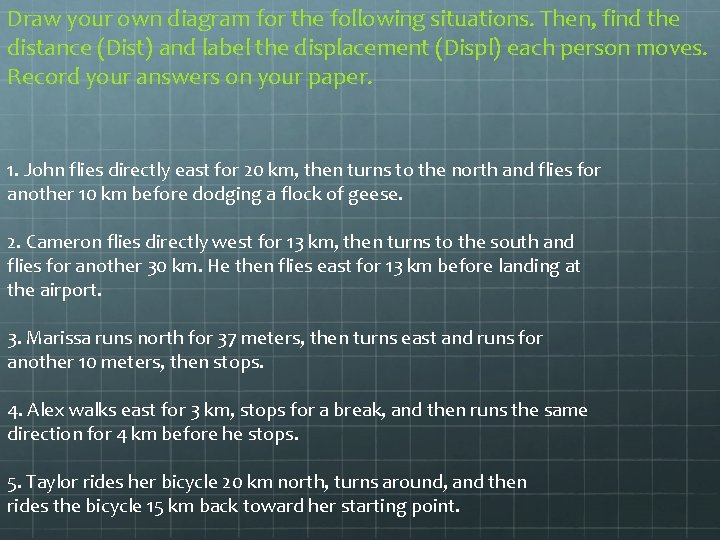Draw your own diagram for the following situations. Then, find the distance (Dist) and label the displacement (Displ) each person moves. Record your answers on your paper. 1. John flies directly east for 20 km, then turns to the north and flies for another 10 km before dodging a flock of geese. 2. Cameron flies directly west for 13 km, then turns to the south and flies for another 30 km. He then flies east for 13 km before landing at the airport. 3. Marissa runs north for 37 meters, then turns east and runs for another 10 meters, then stops. 4. Alex walks east for 3 km, stops for a break, and then runs the same direction for 4 km before he stops. 5. Taylor rides her bicycle 20 km north, turns around, and then rides the bicycle 15 km back toward her starting point.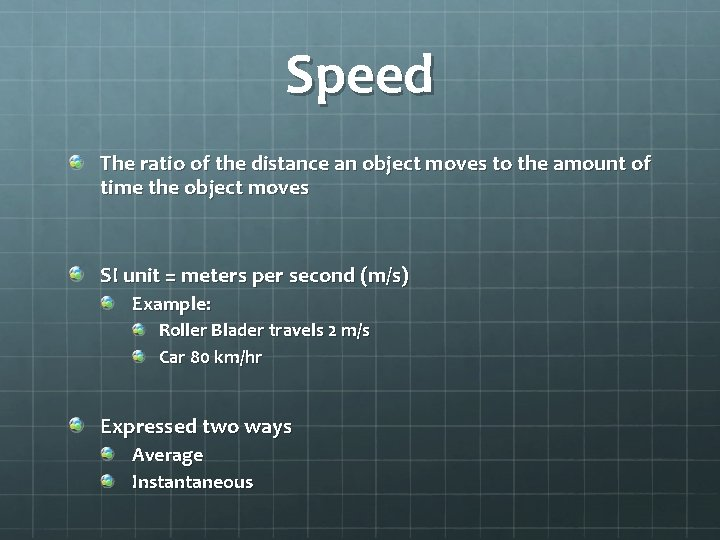Speed The ratio of the distance an object moves to the amount of time the object moves SI unit = meters per second (m/s) Example: Roller Blader travels 2 m/s Car 80 km/hr Expressed two ways Average Instantaneous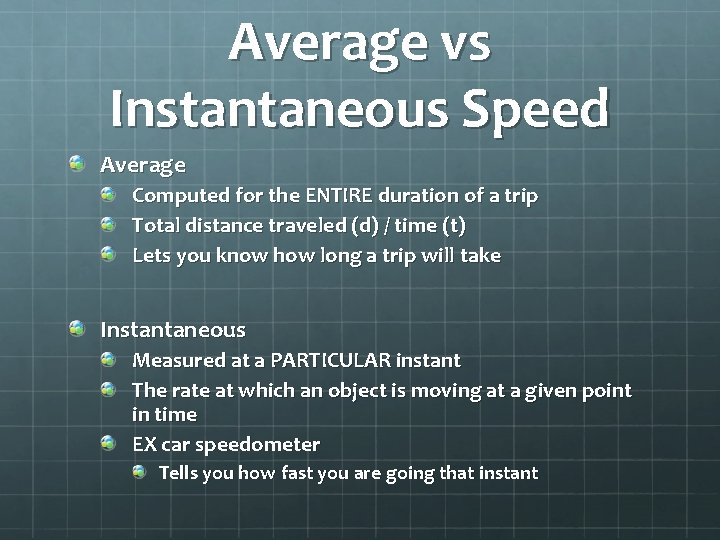Average vs Instantaneous Speed Average Computed for the ENTIRE duration of a trip Total distance traveled (d) / time (t) Lets you know how long a trip will take Instantaneous Measured at a PARTICULAR instant The rate at which an object is moving at a given point in time EX car speedometer Tells you how fast you are going that instant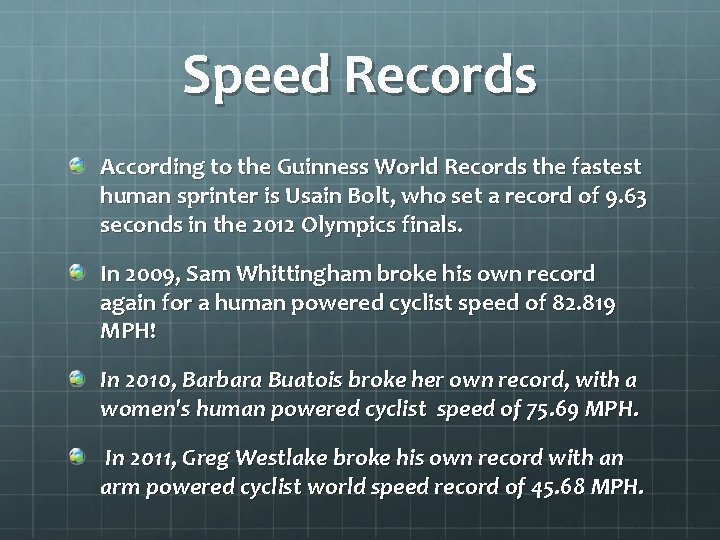Speed Records According to the Guinness World Records the fastest human sprinter is Usain Bolt, who set a record of 9. 63 seconds in the 2012 Olympics finals. In 2009, Sam Whittingham broke his own record again for a human powered cyclist speed of 82. 819 MPH! In 2010, Barbara Buatois broke her own record, with a women's human powered cyclist speed of 75. 69 MPH. In 2011, Greg Westlake broke his own record with an arm powered cyclist world speed record of 45. 68 MPH.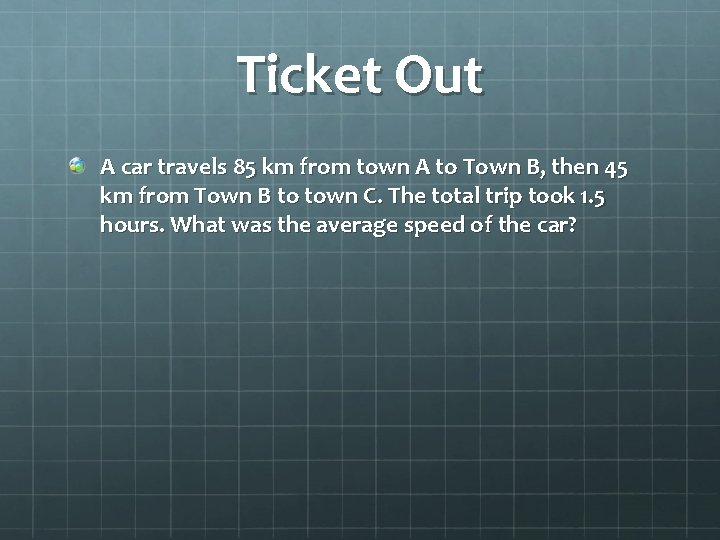Ticket Out A car travels 85 km from town A to Town B, then 45 km from Town B to town C. The total trip took 1. 5 hours. What was the average speed of the car?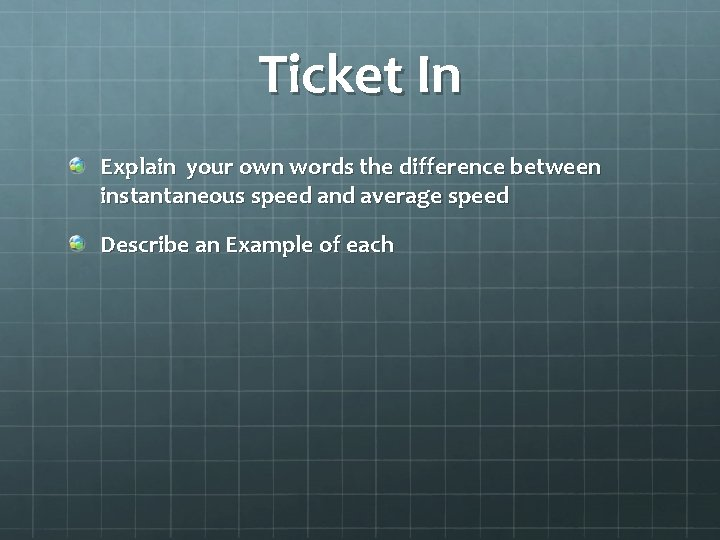Ticket In Explain your own words the difference between instantaneous speed and average speed Describe an Example of each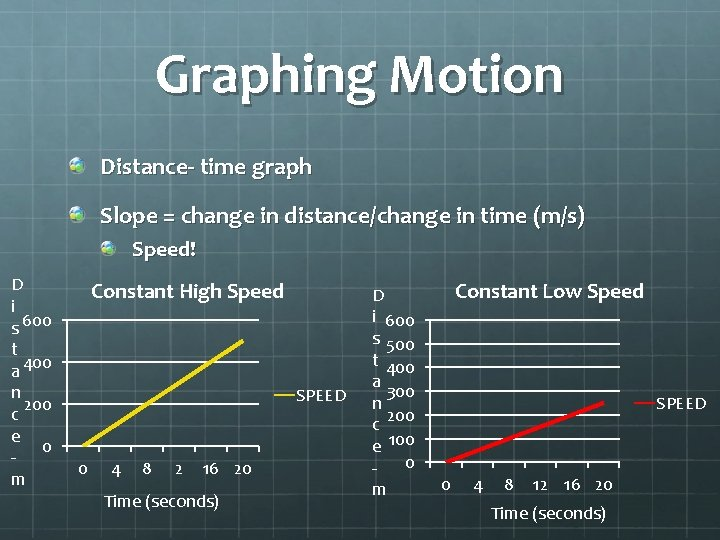Graphing Motion Distance- time graph Slope = change in distance/change in time (m/s) Speed! D i s 600 t a 400 n 200 c e 0 m Constant High Speed SPEED 0 4 8 2 16 20 Time (seconds) D i 600 s 500 t 400 a 300 n 200 c e 100 0 m Constant Low Speed SPEED 0 4 8 12 16 20 Time (seconds)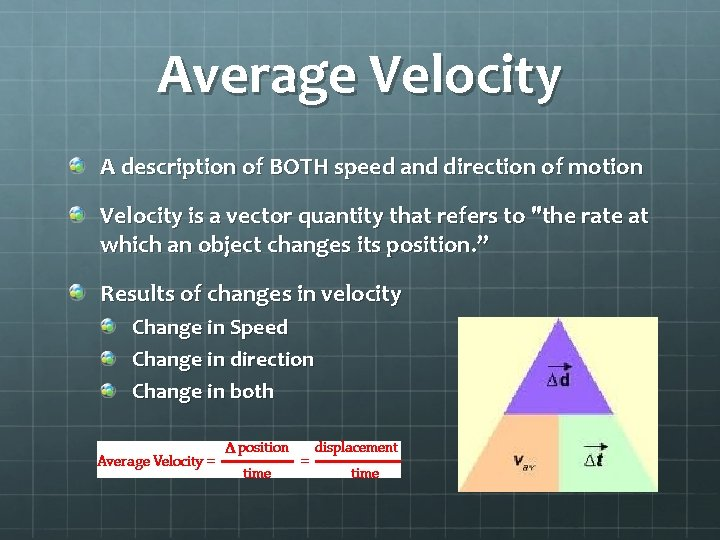Average Velocity A description of BOTH speed and direction of motion Velocity is a vector quantity that refers to "the rate at which an object changes its position. ” Results of changes in velocity Change in Speed Change in direction Change in both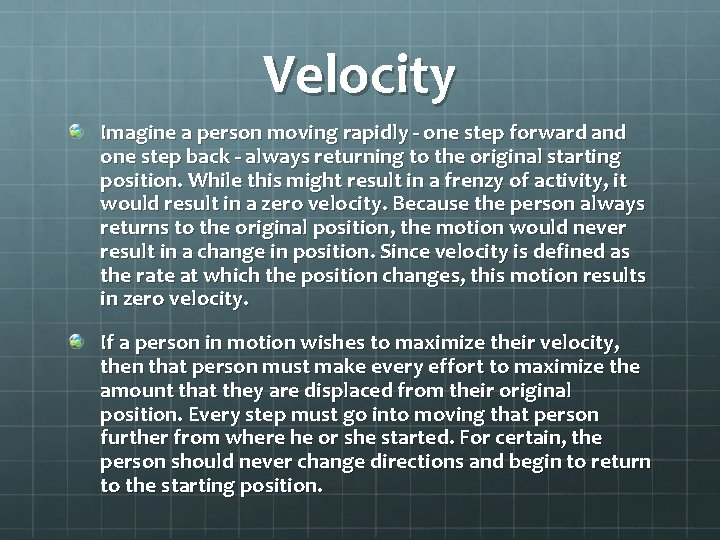Velocity Imagine a person moving rapidly - one step forward and one step back - always returning to the original starting position. While this might result in a frenzy of activity, it would result in a zero velocity. Because the person always returns to the original position, the motion would never result in a change in position. Since velocity is defined as the rate at which the position changes, this motion results in zero velocity. If a person in motion wishes to maximize their velocity, then that person must make every effort to maximize the amount that they are displaced from their original position. Every step must go into moving that person further from where he or she started. For certain, the person should never change directions and begin to return to the starting position.Constant Velocity Moving with uniform motion http: //www. physicsclassroom. com/mmedia/kinema/c pv. cfm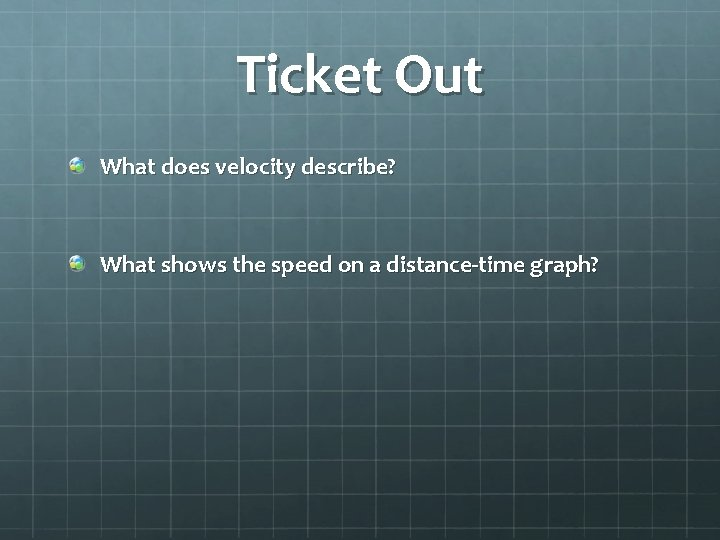Ticket Out What does velocity describe? What shows the speed on a distance-time graph?